# Test Prep Plan - Take a practice test

Take this practice test to check your existing knowledge of the course material. We'll review your answers and create a Test Prep Plan for you based on your results.
How Test Prep Plans work
1
2Based on your results, we'll create a customized Test Prep Plan just for you!
3Study smarter
Study more effectively: skip concepts you already know and focus on what you still need to learn.

# Trigonometric Functions: Tutoring Solution Chapter Exam

Exam Instructions:

Choose your answers to the questions and click 'Next' to see the next set of questions. You can skip questions if you would like and come back to them later with the "Go To First Skipped Question" button. When you have completed the practice exam, a green submit button will appear. Click it to see your results. Good luck!

### Page 1

#### Question 2 2. What is the following radian measure in degrees?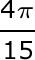### Page 2

#### Question 6 6. In the pictured triangle, side b is 12 and side c is 16. If ∠A is 84 degrees, then approximately how long is side a? Round the answer to the nearest whole number.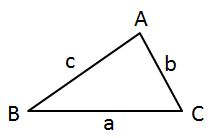#### Question 8 8. In the pictured triangle, side a is 17 and side c is 20. If ∠B is 19 degrees, then approximately how long is side b? Round the answer to the nearest whole number.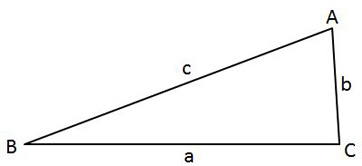#### Question 9 9. What is x?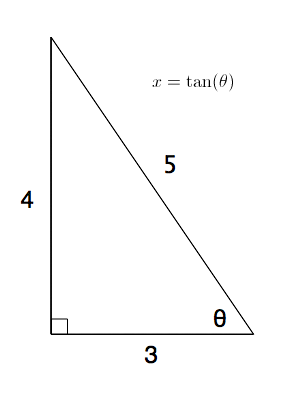#### Question 10 10. In the pictured triangle, side a is 32, side b is 24, and side c is 37. What is the approximate measure of ∠C? Round the answer to the nearest whole number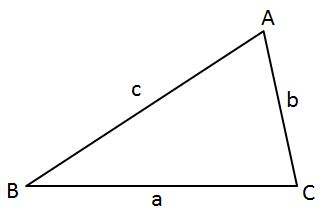### Page 3

#### Question 13 13. In the pictured triangle, side a is 9 and side b is 6. If ∠C is 26 degrees, then approximately how long is side c? Round the answer to the nearest whole number.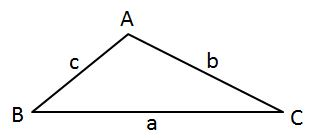#### Question 15 15. In the pictured triangle, ∠A is 62 degrees and ∠C is 86 degrees. If side a is 112 units long, approximately how long is side c?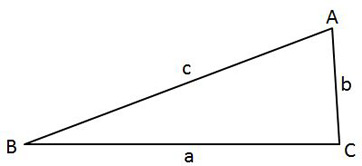### Page 4

#### Question 16 16. Simplify the following expression. Report the answer in degrees.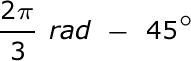#### Question 17 17. In the pictured triangle, ∠A is 137 degrees and ∠B is 28 degrees. If side b is 71 units long, approximately how long is side a?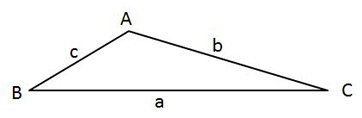#### Question 18 18. In the pictured triangle, ∠A is 98 degrees and ∠B is 12 degrees. If side a is 84 units long, approximately how long is side b?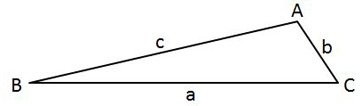### Page 5

#### Question 24 24. What is x?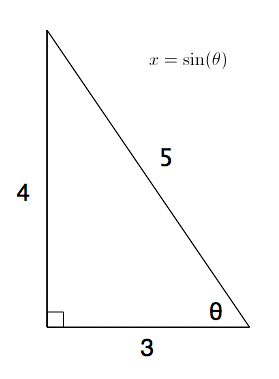### Page 6

#### Question 27 27. Which of these angles can replace x in tan (x)?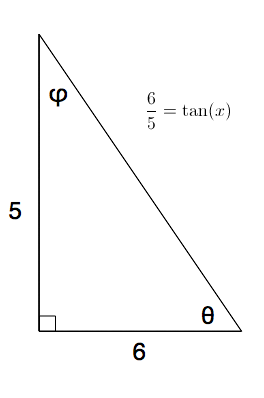#### Question 28 28. In the pictured triangle, side a is 23, side b is 18, and side c is 16. What is the approximate measure of ∠C? Round the answer to the nearest whole number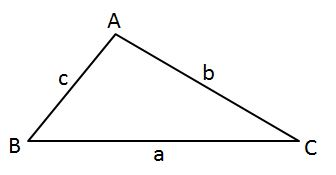#### Question 29 29. In the pictured triangle, ∠A is 81 degrees and ∠B is 67 degrees. If side a is 34 units long, approximately how long is side b?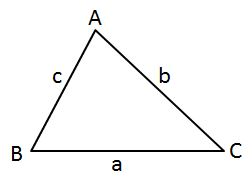#### Trigonometric Functions: Tutoring Solution Chapter Exam Instructions

Choose your answers to the questions and click 'Next' to see the next set of questions. You can skip questions if you would like and come back to them later with the "Go To First Skipped Question" button. When you have completed the practice exam, a green submit button will appear. Click it to see your results. Good luck!

Support